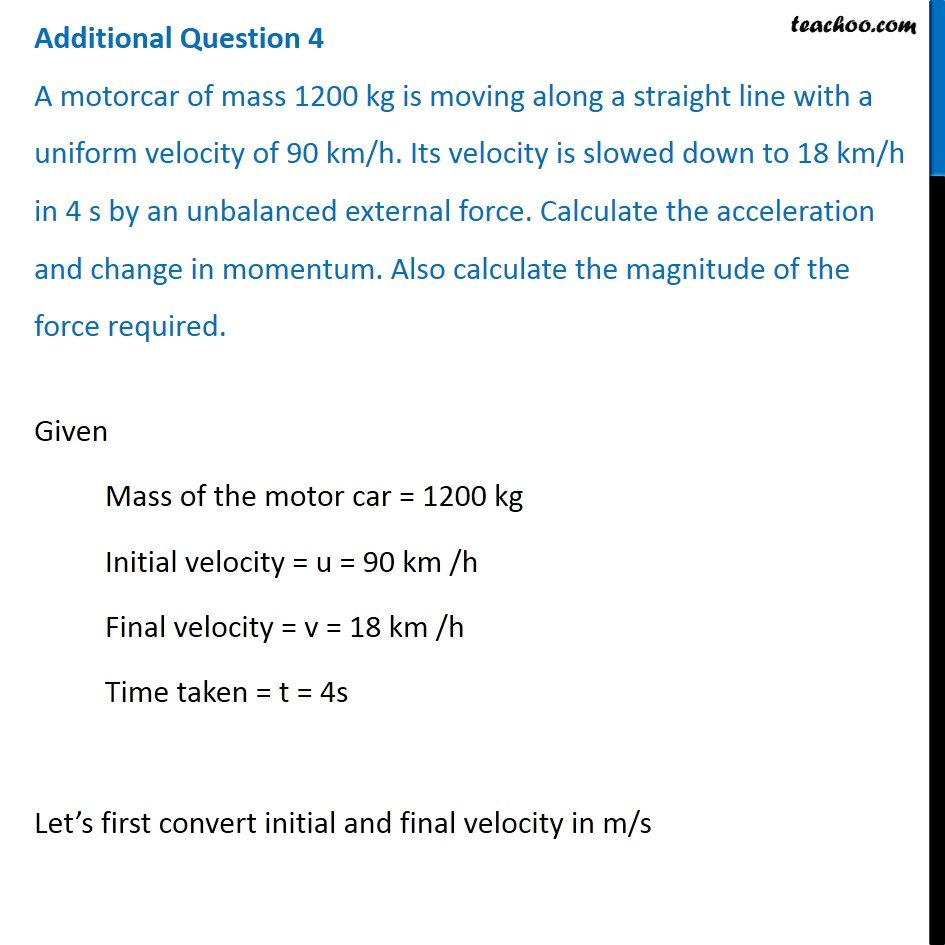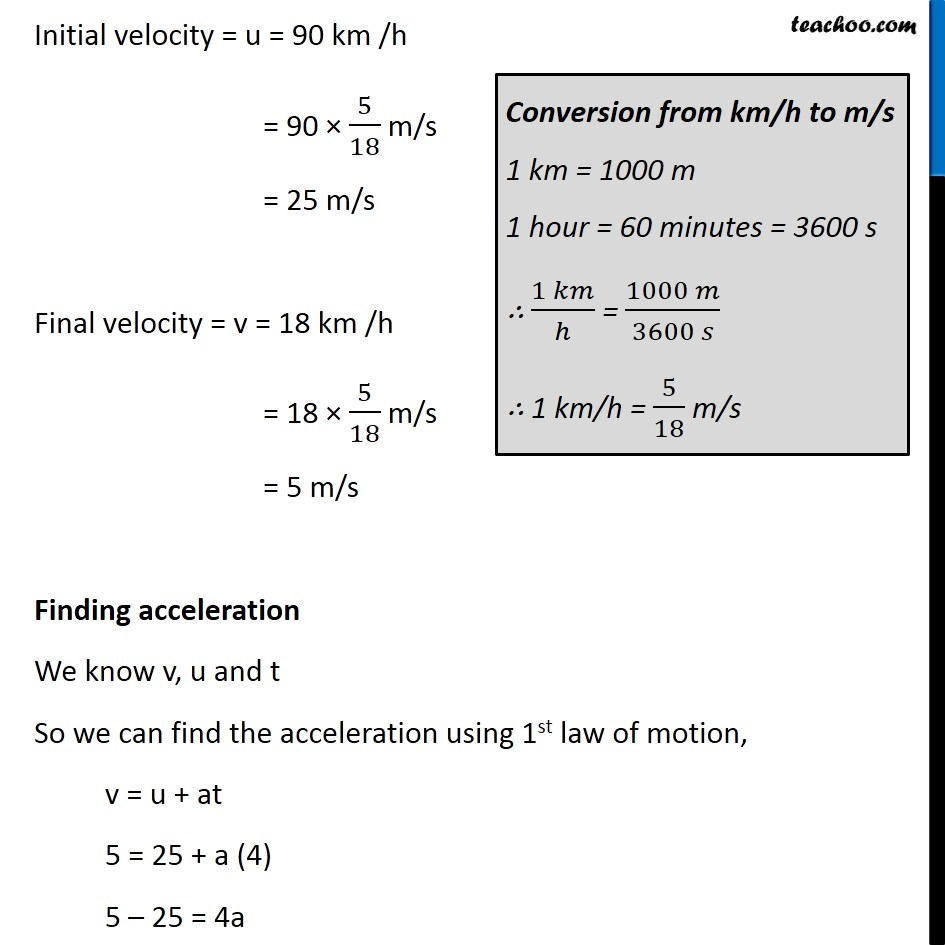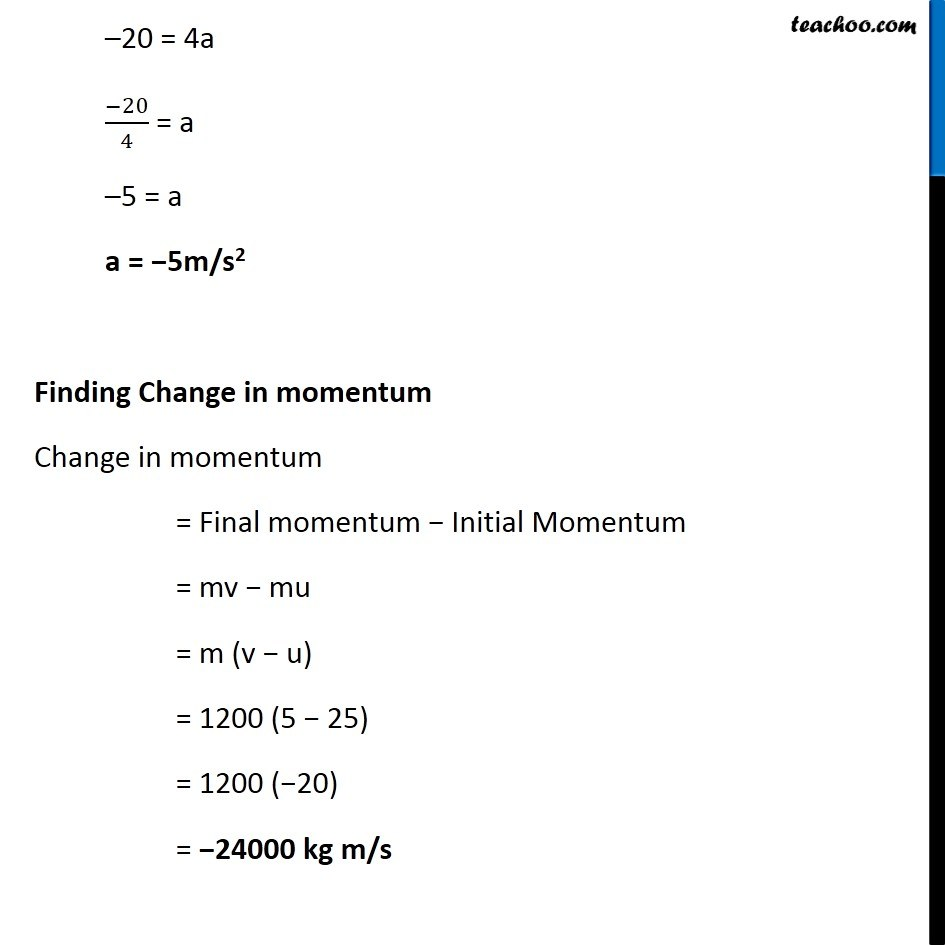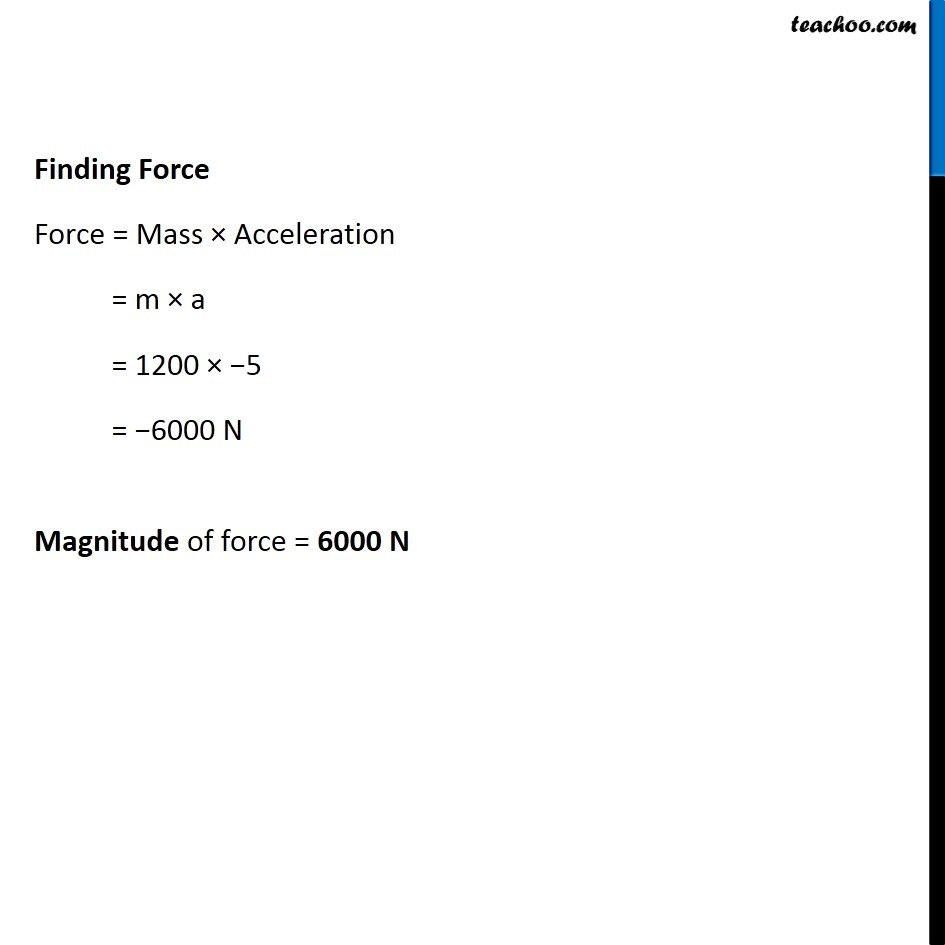Class 9
Chapter 9 Class 9 - Force and Laws Of Motion### Transcript

Additional Question 4 A motorcar of mass 1200 kg is moving along a straight line with a uniform velocity of 90 km/h. Its velocity is slowed down to 18 km/h in 4 s by an unbalanced external force. Calculate the acceleration and change in momentum. Also calculate the magnitude of the force required. Given Mass of the motor car = 1200 kg Initial velocity = u = 90 km /h Final velocity = v = 18 km /h Time taken = t = 4s Let’s first convert initial and final velocity in m/s Initial velocity = u = 90 km /h = 90 × 5/18 m/s = 25 m/s Final velocity = v = 18 km /h = 18 × 5/18 m/s = 5 m/s Finding acceleration We know v, u and t So we can find the acceleration using 1st law of motion, v = u + at 5 = 25 + a (4) 5 – 25 = 4a Conversion from km/h to m/s 1 km = 1000 m 1 hour = 60 minutes = 3600 s ∴ (1 𝑘𝑚)/ℎ = (1000 𝑚)/(3600 𝑠) ∴ 1 km/h = 5/18 m/s –20 = 4a (−20)/4 = a –5 = a a = −5m/s2 Finding Change in momentum Change in momentum = Final momentum − Initial Momentum = mv − mu = m (v − u) = 1200 (5 − 25) = 1200 (−20) = −24000 kg m/s Finding Force Force = Mass × Acceleration = m × a = 1200 × −5 = −6000 N Magnitude of force = 6000 N